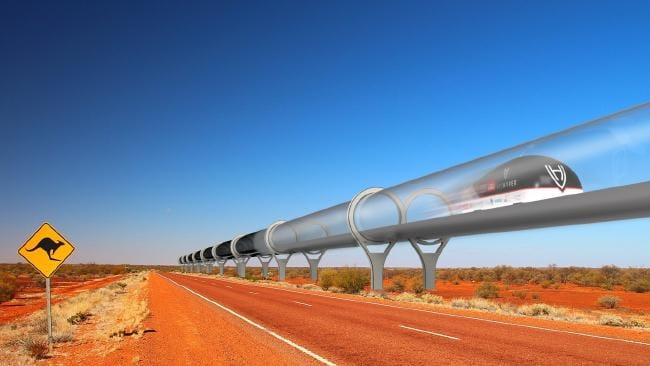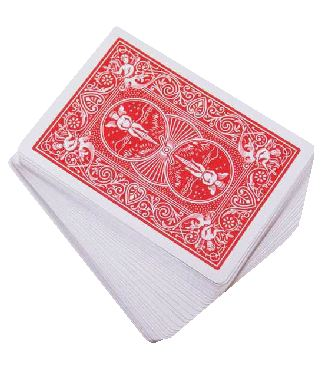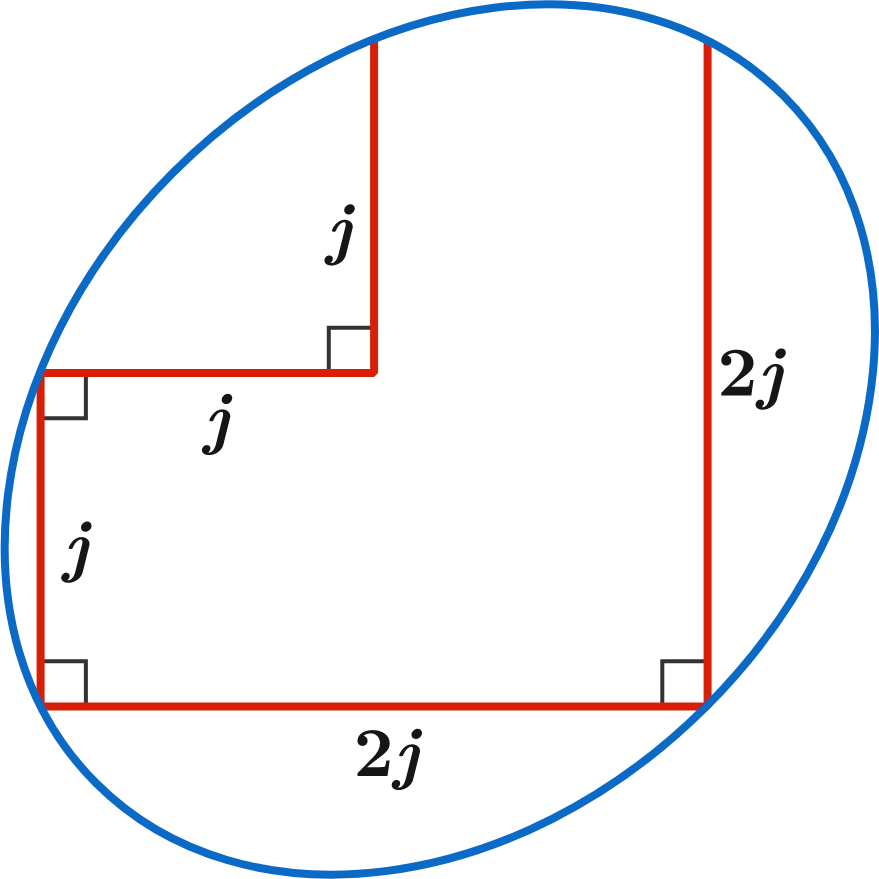# Problems of the Week

Contribute a problem

What is the value of $n$ such that $\displaystyle {\large \sum_{a_1=0}^4 \sum_{a_2=0}^{a_1} \cdots \sum_{a_n=0}^{a_{n-1}} 1} = 1001 ?$

A recent proposal for rapid inter-city transit is the Hyperloop, popularized by Elon Musk in 2012. The idea is to transport people inside of a levitating pod, by way of a partially evacuated tube running from city to city. Among the advertised benefits are its high speed (close to the speed of sound) and the potential to power the Hyperloop with solar energy.Inside the tube, the pod enjoys a reduced atmosphere with a pressure of $\SI{0.1}{\kilo\pascal},$ about $\frac{1}{1000}^\text{th}$ the pressure at sea level. Thanks to the evacuated tunnel and the magnetic levitation used to lift and propel the train, the energy needed to maintain the train's cruise velocity against the opposing forces of drag is very low.

However, this boon requires an up-front energy expenditure in the form of evacuating a $\SI{600}{\kilo\meter}$-long tunnel large enough to fit the train.

Approximately how many one-way trips of a comparable open-air (i.e. not in a tube), high-speed train would it take to recoup the initial energy expenditure to draw the vacuum?

Assume

• the pods and the Hyperloop tube have a radius of $r = \SI{2.5}{\meter},$ the density of air is $\rho_\text{air} = \SI[per-mode=symbol]{1.22}{\kilo\gram\per\meter\cubed},$ the distance from Los Angeles to San Francisco is $d_\text{LA to SF} \approx \SI{600e3}{\meter},$ the drag coefficient is $C_\text{D} \approx 0.15,$ and when run as a high-speed train, the pods travel at $\SI[per-mode=symbol]{150}{\meter\per\second};$
• once drawn, the Hyperloop tube maintains the partial vacuum state.In group theory, if you take a standard deck of cards and shuffle it exactly the same way repeatedly, you will eventually get back to the original order of the cards. For a standard deck of 52 cards, this could take many deck shufflings!

However, what if your deck consisted of only 8 cards?

With only 8 cards, what is the smallest number of identical shufflings you need to make in order to be guaranteed to return to the original order of the cards (somewhere along the way)?

Assumption: All shuffles are identical. For example, if the first shuffle takes the third card to the fifth position, then all successive shuffles will also take the third card to the fifth position. Or, more generally, for all $n$, if the $n^\text{th}$ card went to the $m^\text{th}$ position, then the $n^\text{th}$ card would necessarily go to the $m^\text{th}$ position on all successive shufflings.

Clarification:. You don't get to pick the way the cards are shuffled, but they must be shuffled the same way every time.In the ellipse shown, let $a$ and $b$ be the lengths of the semi-major and semi-minor axes, respectively.

What is the value of $j$ for which $a^2 + b^2 = 19200?$

$1,1,2,3,5,8,13,21,...$

If we divide the terms in the Fibonacci sequence above by $2$, then the remainders will be $1,1,0,1,1,0,1,1,0,...$.
Obviously, the ratio of the numbers of $0$s and $1$s in the sequence goes to $1:2.$

If we divide the terms by $3$, then the remainders will be $1,1,2,0,2,2,1,0,1,1,2,0,...$.
The ratio of the numbers of $0$s, $1$s, and $2$s in the sequence now goes to $2:3:3.$

Now, we divide the terms by a positive integer $n$ and obtain a sequence of remainders.
Then the ratio of the numbers of $0$s, $1$s, $2$s, $\ldots,$ $(n-1)$s in the sequence goes to $1:1:1:\ldots:1.$

What is the sum of all possible $\frac1n,$ if $n$ can also be 1?

Clarification: Each whole number smaller than $n$ has the same probability to appear in the sequence of remainders. This is also the reason that $n$ can also be $1$.

×